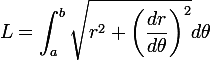# Calculate number of turns in Archimedes spiral

• evilbrent

#### evilbrent

Hi,

I'm an engineer designing a spring system for a garage roller door. I need to know the number of turns of the door for all the size combinations.

I've found this page which gives a good equation for finding the length if you know the number of turns, starting radius and gap between spirals:The equation of the spiral is r=x+yθ, so x=starting radius, y=gap/2∏, and to find L we're taking the integral from a=0 to b=2∏n (where n=turns).

When you know n, this is straightfoward, and even I could work that out. But it's been a decade since I've done anything like this, so I was wondering if anyone could help me find an expression for n in this:

L=$^{2∏n}_{0}$ $\sqrt{(a+bθ)^2+b^2}dθ$

Lord help me, my way of solving this is to find L for n=1,2,3,4,5 etc, graph it in excel and use "find trendline" to get an equation. Any help appreciated, thanks.

Last edited:

Hey!

If you're only looking for the answer, you can use this.

For methodology, you can do two substitutions: first $v=a+b\theta$

Then you need to make a second substitution v=b*sinh(u). Don´t forget the derivatives. For more information you can visit this topic: